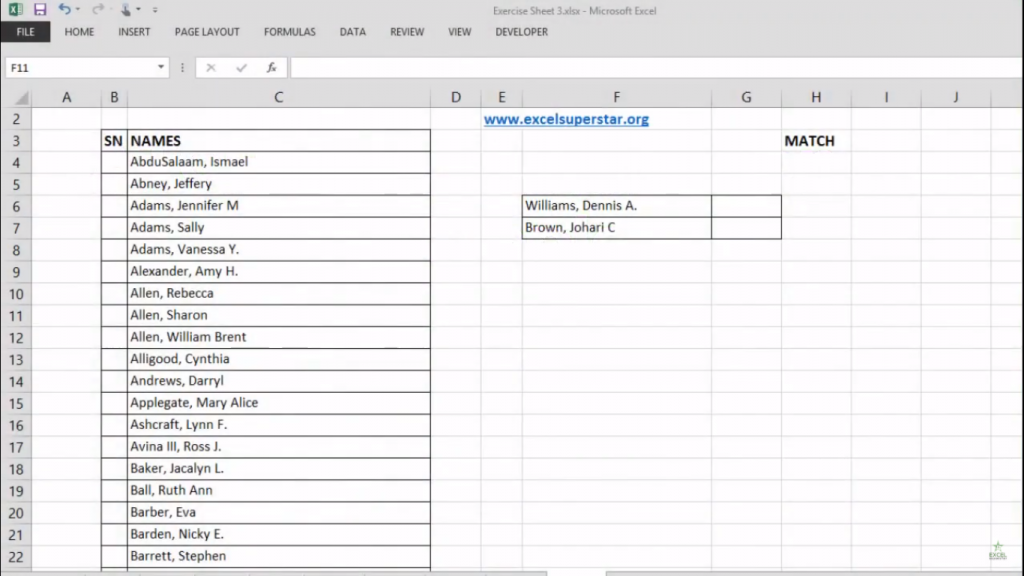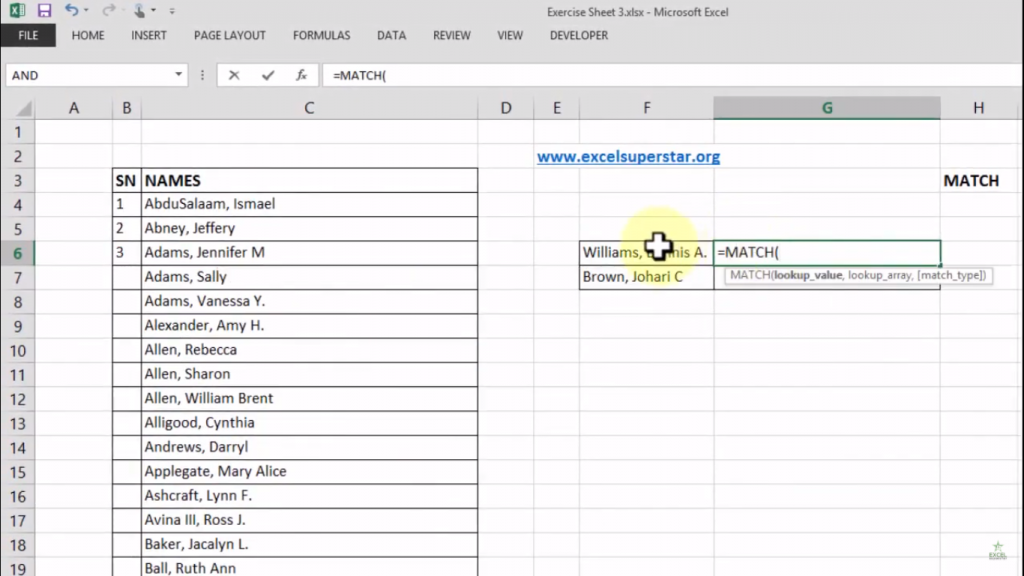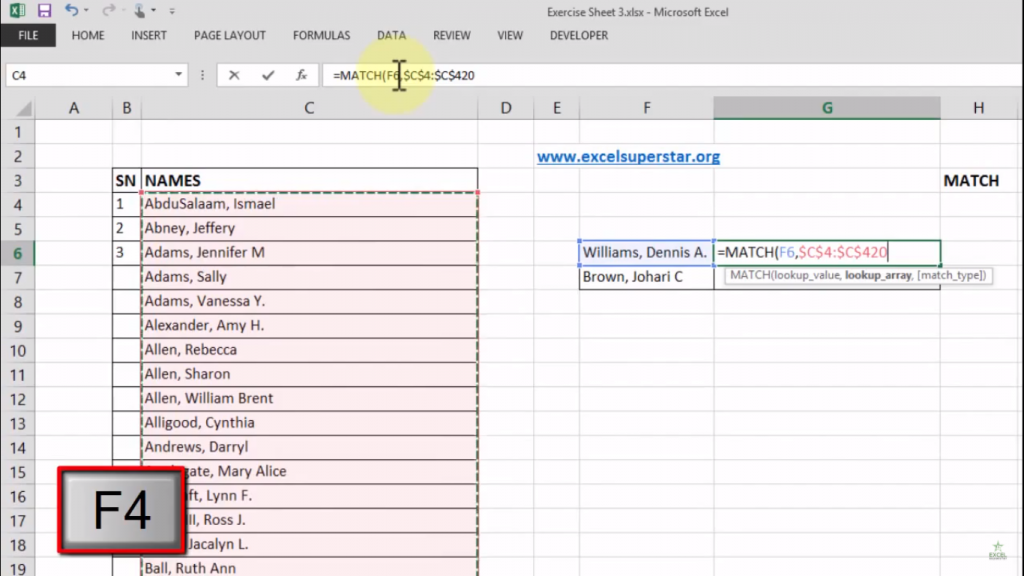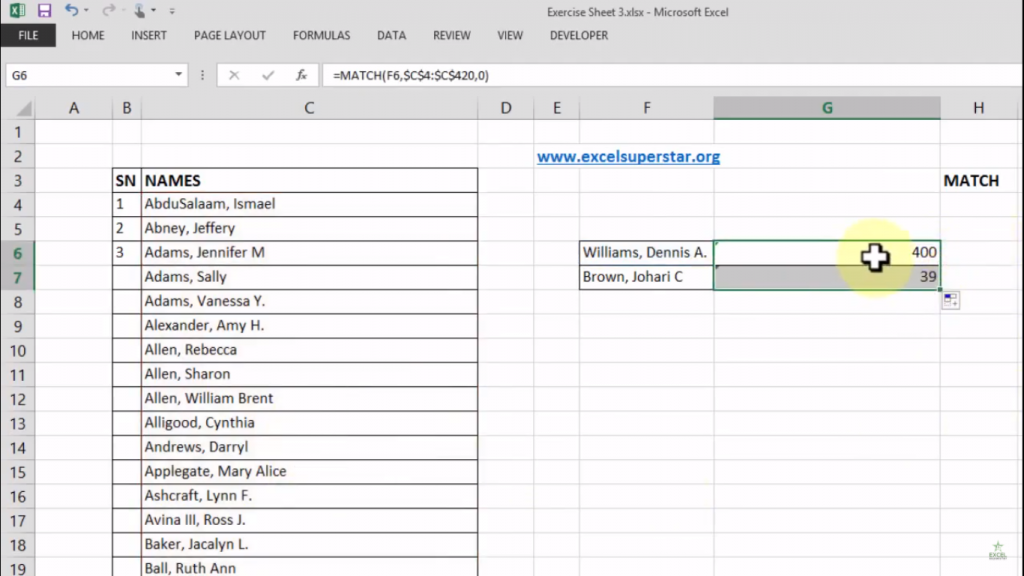# Excel Match FormulaMatch Formula in Excel is a must-know formula in order to carry out the position of the names in Excel whether it is vertically or horizontally. But many people do not know how to do it.

So in this tutorial, I will be telling you about the basics of Match Formula in Excel so that you can carry out the position of the names in Excel easily without any trouble.50% OFF on Excel Course

• Get 2-Year Access with unlimited views of 220+ videos
• Use Coupon code - NAV50OFF & Start learning

ExampleThere is a list of 417 unique names that are not repeated on the right side there are 2 names given. Now what I want is that I want to know the position of the 2 names from this 417 unique names list.

So to know the position of these 2 names we will be applying Match Formula in Excel. Let us how to apply it :

1. Write =MATCH(There will be 3 parameters containing lookup value, lookup array, and match-type. So here lookup value means the name column so to choose the name column what we will do is:

2. Choose lookup value as the name column so the formula will be =MATCH(F6,

Press Comma after every function

Now lookup array means the list of entire names so to choose the entire list of names what we will do is:

3. Select a cell and press the Shortcut Key Shift + Ctrl + Down Arrow Key

4. Then press F4 so the formula will be =MATCH(F6, \$C\$4:\$C\$C420,Lastly, match type means to carry out the exact match of an answer which means if the name is exact then only my answer will be considered as an exact match. So to find an exact match what we will do is:

5. Write 0 and close the bracket so the formula will be =MATCH(F6, \$C\$4:\$C\$C420,0)

6. Lastly, press Enter

So as you copy-paste the names and drag it to the bottom you will see the position of these 2 names which we were looking for that is 400 and 39Conclusion:

So Match Formula in Excel only shows you the position either vertically or horizontally. But keep in mind that it is a Junior Formula which means that it cannot take the table array it can take only as a part of a column or as a part of an array.

So keep practicing this Match Formula and if you have any doubt then do let us know in the comment section below. I will be glad to help you out.